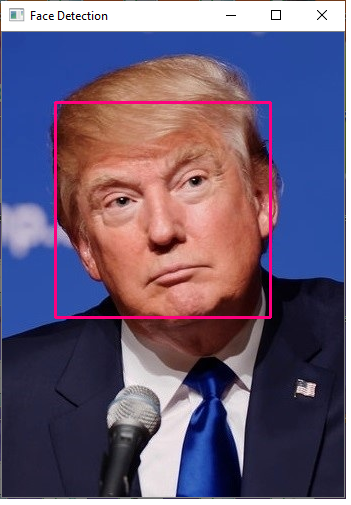﻿# How to Detect Faces in an Image in Python using OpenCVIn this article, we show how to detect faces in an image in Python using the OpenCV module.

Using computer vision software such as OpenCV, we can create a face classifier using the cv2.CascadeClassifier() function.

We then create an array of all the faces in the image.

We then loop through each of the faces in the array and draw a rectangle around each of them.

Let's look at some examples below first with a single face and then another with multiple faces.

So below is a picture with Donald Trump.After running our code on this image, this produces the image below.In the same way that we detected a single face in our image, we can do the same with multiple faces.

Below is an image of Mike Pence and his wife.After applying our code, we get the following output image shown below.So let's now go over the code so that we can see all that is necessary to detect faces.

Let's now go over this code.

First, we import OpenCV using the line, import cv2

Next, we read in the image, which in this case is, Donald-Trump.png

We then create a grayscale version of the image.

We then create a variable, face_classifier, which stores an XML file that allows for a face classifier. You can download this file at the following link: haarcascade_frontalface_default.xml

We then create a variable, faces, which we set equal to the detectMultiScale() function. This function detects the areas where faces are found in the image. In this way, the faces variable functions as an array, storing all the areas of faces in the image.

Now, in order to draw rectangles around each of the faces, we use a for loop that loops through each of the items in the faces array. We use the cv2.rectangle() function to draw a red rectangle around eahc face in the image.

We then show the image.

And this is one method to detect faces in Python using computer vision software such as OpenCV.

For basic, simple images, the software is very good at identifying faces. For more complex images, where faces may not be easily identifiable or are complex in nature, the software may not make correct identifications.

So this is a bais method to detect faces in an image in Python using OpenCV.

Related Resources